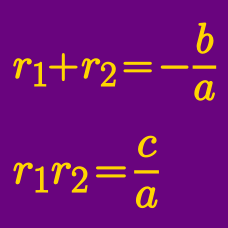Algebra

# Vieta's Formula: Level 5 Challenges

If the roots of $p(x) = x^3 + 3x^2 + 4x - 8$ are $\color{#D61F06}{a}$, $\color{#3D99F6}{b}$ and $\color{#69047E}{c}$, what is the value of

$\color{#D61F06}{a}^2 \big(1 + \color{#D61F06}{a}^2\big) + \color{#3D99F6}{b}^2 \big(1 + \color{#3D99F6}{b}^2\big) + \color{#69047E}{c}^2 \big(1 + \color{#69047E}{c}^2\big)?$

If $\alpha , \beta , \gamma$ are roots of the equation $x^{3} + 3x + 9 = 0,$ find the value of $\alpha^{9} + \beta^9 + \gamma^{9}.$

Consider all pairs of non-zero integers $(a,b)$ such that the equation

$( ax-b)^2 + (bx-a)^2 = x$

has at least one integer solution.

The sum of all (distinct) values of $x$ which satisfy the above condition can be written as $\frac{ m}{n}$, where $m$ and $n$ are coprime positive integers. What is the value of $m + n$?

Let $x_1,x_2,\ldots,x_{2015}$ be the roots of the equation $x^{2015} + x^{2014} + x^{2013} + \ldots + x^2 + x + 1 =0.$ Evaluate

$\frac1{1-x_1} + \frac1{1-x_2} + \ldots + \frac1{1-x_{2015}}.$

What is the largest integer $n \leq 1000$, such that there exist 2 non-negative integers $(a, b)$ satisfying

$n = \frac{ a^2 + b^2 } { ab - 1 } ?$

Hint: $(a,b) = (0,0)$ gives us $\frac{ 0^2 + 0^2 } { 0 \times 0 - 1 } = 0$, so the answer is at least $0 .$

×